Statistics

# Confidence Intervals: Statistical Techniques

by Liz Roth-Johnson, Ph.D.

You may not think of beer brewing as a scientific pursuit, but consider how many variables must be carefully controlled in order to reproducibly brew the same beer with the right appearance, taste, and aroma. Small differences in the quality of the raw ingredients, the temperature of the brew, or the precise way in which microorganisms break down sugars to produce alcohol could have noticeable effects on the final beverage. If you were managing a brewery and needed to send a consistently crafted beer to market, would you be content leaving all of these different brewing variables up to chance?

Because of the complicated nature of brewing, beer companies have a long history of employing scientists to help them analyze and perfect their beers. In 1901, the Guinness Brewery in Dublin, Ireland, established its first official research laboratory. Guinness employed several young scientists as beer makers. These scientists-turned-brewers applied rigorous experimental approaches and analytical techniques to the process of brewing (Figure 1).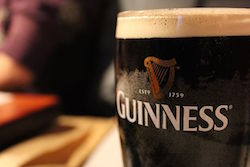Figure 1: The process of beer making is a surprisingly scientific pursuit. The Guinness Brewery founded its first research laboratory in 1901 and hired a young scientist who made a lasting impact on the field of statistics.image © Morabito92

To see what this might have looked like, let’s imagine a scenario in which one of Guinness’ scientific brewers is working on a new quality control procedure. He (as the vast majority of beer workers at the time were men) is recording a variety of quantitative measurements to be used as benchmarks throughout the brewing process.

Today he is analyzing a set of density measurements taken on the tenth day of brewing from five different batches of the same type of beer (Figure 2). From this dataset, the brewer would like to establish a range of density measurements that can be used to assess the quality of all future beer batches on the tenth day of brewing.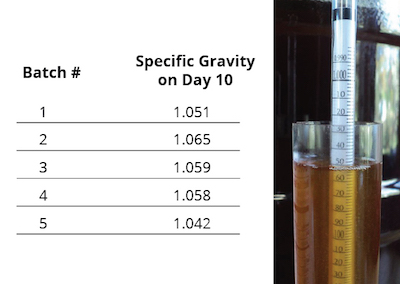Figure 2: Measurements of beer density recorded for five batches of the same type of beer on the tenth day of brewing. Beer density is reported as specific gravity, which is the density of the beer divided by the density of water. Specific gravity is typically measured with a hydrometer, pictured on the right. The higher the hydrometer floats, the higher the density of the fluid being tested.image © Schlemazl (hydrometer)

As we will see in this module, the brewer’s analysis would benefit greatly from the techniques of statistical inference. When studying a procedure like brewing, the statistical population includes all possible observations of the procedure in the past, present, and future. Because of the impracticality of studying the entire population, the brewer must instead use a smaller subsample, such as the data presented above, to make inferences about the entire population. Here we will highlight how one technique, the confidence interval, can be used to estimate a parameter from a subsample dataset. (To review the relationship between subsamples and populations, or to learn more about inferential statistics in general, see Introduction to Inferential Statistics.)

## In pursuit of the perfect brew

The histories of science and beer are surprisingly intertwined. Before the development of modern science, ancient brewers experimented through trial-and-error, testing different combinations of ingredients and brewing conditions to concoct palatable beverages. In later centuries, several important scientific advancements occurred in connection with brewing. For example, James Prescott Joule conducted some of his classic thermodynamics experiments in his family’s brewery where he had access to high-quality thermometers and other useful equipment (see Energy: An Introduction and Thermodynamics I); biochemist Sören Sörensen invented the pH scale while working for Carlsberg, a Danish brewing company (see Acids and Bases: An Introduction); and one of the most widely used tools used in inferential statistics was developed by William Sealy Gosset, a chemist working for Guinness.

Identifying the relationship between a subsample and a population is a key concept at the heart of inferential statistics. However, at the turn of the twentieth century, statisticians did not differentiate between subsample statistics and population parameters. Karl Pearson, one of the great statisticians of the time, typically worked with such large subsamples that any difference between the subsample statistics and population parameters would, in theory, be negligibly small. But this posed a problem for Gosset, who needed a way to select the best varieties of barley to use in Guinness’ beer by analyzing only very small subsamples of data collected from the farm. After spending a year studying with Pearson, Gosset developed a new mathematical tool that could be used to estimate a population mean based on a small subsample. Because Guinness’ board of directors was eager to protect company secrets, Gosset published his work in 1908 under the pseudonym ‘Student’.

Gosset’s mathematical tool, now known as “Student’s t-distribution,” has become an important component of several inferential statistics techniques used in science, including the construction of a confidence interval. Student’s t-distribution is a probability distribution that looks similar to a normal distribution but with more pronounced tails (Figure 3). The t-distribution can be used to help estimate a population mean when the subsample size is small and the population’s standard deviation is unknown. While the mean of a very large subsample is likely to fall close to the population mean, small subsamples can be more unpredictable. The t-distribution accounts for the inherent uncertainty associated with small subsamples by assigning less probability to the central values of the distribution and more probability to the extreme values at the tails. The larger a subsample becomes, the more the t-distribution looks like a normal distribution; the decision to use a t-distribution rests on the assumption that the underlying population is normally distributed.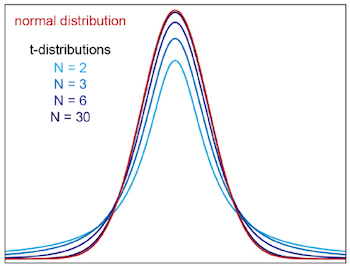Figure 3: Student’s t-distribution looks similar to a normal distribution but has more pronounced tails when subsample sizes are small. Four different t-distributions are shown in varying shades of blue, each of which corresponds to a different subsample size (N). Notice how the t-distribution approaches a normal distribution (shown in red) as the degrees of freedom (i.e., the sample size) becomes larger.

Shortly after Gosset’s original paper was published, Ronald Fisher (whose own work is highlighted in Statistics in Science) further developed and applied the work. In particular, Fisher defined a metric called the “t-score” (or t-statistic). As we will see in a moment, this t-score can be used to construct a confidence interval, especially when subsample sizes are small. In 1937, Polish mathematician and statistician Jerzy Neyman built upon this work and formally introduced the confidence interval more or less as it is used by scientists today.

Comprehension Checkpoint

The mathematical tool developed by Gosset was called Student's t-distribution because

## Confidence in what, exactly?

Confidence intervals use subsample statistics like the mean and standard deviation to estimate an underlying parameter such as a population mean. As the name suggests, confidence intervals are a type of interval estimate, meaning that they provide a range of values in which a parameter is thought to lie. This range reflects the uncertainty related to the estimation, which is largely determined by a confidence level selected at the beginning of the analysis. The higher the confidence level, the less uncertainty is associated with the estimation. For example, a confidence interval calculated at the 95% confidence level will be associated with less uncertainty than a confidence interval calculated at the 50% confidence level.

One common misconception is that the confidence level represents the probability that the estimated parameter lies within the range of any one particular confidence interval. This misconception can lead to false assumptions that the confidence interval itself provides some intrinsic measure of precision or certainty about the location of the estimated parameter. In fact, the confidence level represents the percentage of confidence intervals that should include the population parameter if multiple confidence intervals were calculated from different subsamples drawn from the same population.

To think about what this really means, let’s imagine that you are an environmental scientist studying chemical runoff from a local factory. You have detected lead in a pond near the factory and want to know how the lead has affected a small population of 25 frogs that live in the pond. Although you would like to test the entire population of 25 frogs, you only have five lead testing kits with you. Because of this, you can only test a random subsample of five frogs and then use your data to make inferences about the entire population. After collecting and testing blood samples from five random frogs, you get the following results:

Subsample #1
Frog # Lead in Blood (µg/dL)
1 10.3
2 12.5
3 9.7
4 5.6
5 14.5
Mean = 10.5 µg/dL
Standard deviation = 3.3 µg/dL
95% confidence interval = 10.5 ± 4.2 µg/dL

Using this subsample dataset, you calculate a confidence interval at the 95% confidence level. Based on this interval estimate, you feel reasonably sure that the population mean (in this case the average level of lead in the blood of all 25 frogs in this pond) lies somewhere between 6.4 and 14.7 ug/dL – but what would happen if you repeated this entire process again? Let’s say you go back to the same pond, collect two more random subsamples from the same population of 25 frogs and find the following results:

Subsample #2
Frog # Lead in Blood (µg/dL)
1 12.9
2 12.9
3 15.8
4 10.7
5 16.9
Mean = 13.8 µg/dL
Standard deviation = 2.5 µg/dL
95% confidence interval = 13.8 ± 3.1 µg/dL
Subsample #3
Frog # Lead in Blood (µg/dL)
1 16.9
2 5.6
3 11.0
4 12.9
5 3.7
Mean = 10.0 µg/dL
Standard deviation = 5.4 µg/dL
95% confidence interval = 10.0 ± 6.7 µg/dL

Although all three subsamples were randomly drawn from the same population, they generate three different 95% confidence interval estimates for the population mean (Figure 4). Perhaps most notable is the difference in size among the three confidence intervals. Subsample 3, for example, has a much larger confidence interval than either of the other two samples. Subsample 3 also has the most variation, or spread, among its five data points, which is quantified by its particularly large standard deviation. Greater variation within a subsample leads to a greater degree of uncertainty during the estimation process. As a result, the range of a confidence interval or other statistical estimate will be larger for a more varied sample compared to a less varied sample even when both estimates are calculated at the same confidence level.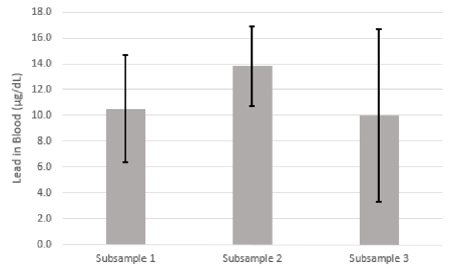Figure 4: Different subsamples generate different confidence intervals, even when randomly selected from the same population. Each subsample confidence interval is represented by black error bars. In this case, subsamples 1 and 3 generate confidence intervals that include the population mean (10.1 ug/dL) while subsample 2 does not. At the 95% confidence level, we would expect 95 out of every 100 subsamples drawn from the same population to generate a confidence interval that includes the population parameter of interest.

Because this is an illustrative example, it turns out that we know the actual parameters for the entire 25-frog population—a luxury we would not normally have in this kind of situation. The population mean we have been trying to estimate is in fact 10.1 µg/dL. Notice that the second subsample’s confidence interval, despite being at the 95% confidence level, does not include the population mean (Figure 4). If you were to collect 100 different random subsamples from the same frog population and then calculate 100 different confidence intervals based on each subsample, 95 out of the 100 subsamples should generate confidence intervals that contain the population parameter when calculated at the 95% confidence level. When calculated at the 50% confidence level, only 50 out of the 100 subsamples would be expected to generate confidence intervals that contain the population parameter. Thus, the confidence level provides a measure of probability that any particular subsample will generate a confidence interval that contains the population parameter of interest.

In practice, confidence intervals are generally thought of as providing a plausible range of values for a parameter. This may seem a little imprecise, but a confidence interval can be a valuable tool for getting a decent estimation for a completely unknown parameter. At the beginning of the frog scenario above, we knew absolutely nothing about the average level of lead in the population before analyzing any one of the three subsamples. In this case, all three subsamples allowed us to narrow down the value of the population mean from a potentially infinite number of options to a fairly small range. If, on the other hand, we had wanted to precisely pinpoint the population mean, then the analysis would not have been nearly as helpful. When dealing with confidence intervals—as with any statistical inference technique—it is ultimately up to researchers to choose appropriate techniques to use and ascribe reasonable meaning to their data (for more about deriving meaning from experimental results, see Introduction to Inferential Statistics).

Comprehension Checkpoint

The higher the confidence level,

## Constructing a confidence interval

To see how a confidence interval is constructed, we will use the brewer’s density dataset from the beginning of the module (Figure 1). This dataset gives us a subsample with a mean of 1.055 and a standard deviation of 0.009. (See Introduction to Descriptive Statistics for more information about calculating mean and standard deviation). To differentiate these subsample statistics from the population parameters (where µ represents the population mean and σ the standard deviation), it is common practice to use the variables m and s for the subsample mean and standard deviation, respectively. The size of our subsample (N) is 5. In the four steps below, we will use these values to construct a confidence interval for the population mean to answer our brewer’s original question: What is the average density of this beer on the tenth day of brewing?

### Step 1: Select a confidence level

First, we need to choose a confidence level for our calculation. A confidence level can be any value between, and not including, 0% and 100% and provides a measure of how probable it is that our interval estimate includes the population mean. In theory, any confidence interval can be chosen, but scientists commonly choose to use 90%, 95%, or 99% confidence levels in their data analysis. The higher the value, the larger the confidence level and the more probable it is that the confidence interval will include the actual population mean. For our calculation, we will choose a 95% confidence level.

### Step 2: Find the critical value

The next step is to find the “critical value” that corresponds with our sample size and chosen confidence level. A critical value helps us to define the cut-off regions for the chosen test's statistics where the null hypothesis can be rejected. We begin by calculating a value called alpha (α), which is determined by our chosen confidence level using the equation:

$\alpha =1-\frac{\text{confidence level}}{\text{100%}}$

For a confidence level of 95%, alpha equals 0.05. We can now use our subsample size and alpha value to use a look-up table or online calculator to find the critical value. Because our subsample size is quite small (N = 5) and we know nothing about the variation of beer density among the entire population, we will express our critical value as a t-score. The t-score can be found using a lookup table like the one shown in Figure 5. Typically a t-score lookup table will organize t-scores by two metrics: the "cumulative probability" and the "degrees of freedom." Cumulative probability helps us to determine if a random variable's value falls within a specific range; the degrees of freedom are the number of observations in a sample that are free to vary when making estimations from subsample data.

• The cumulative probability (p) is calculated using alpha: p = 1 – α/2. Because our alpha is 0.05, the cumulative probability we are interested in is 0.975.
• The degrees of freedom is the subsample size minus one: N – 1. Because our subsample size is 5, the degrees of freedom equals 4.

Using the lookup table, we now want to find where our cumulative probability (0.975) intersects with the degrees of freedom (4). As shown in Figure 5, this leads us to the t-score 2.776. This is our critical value.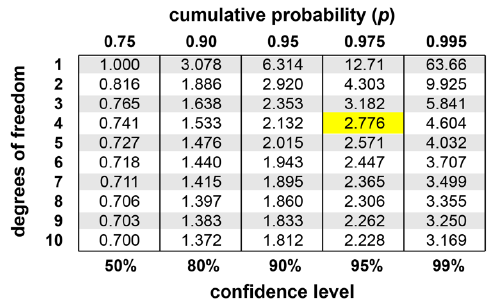Figure 5: A t-score lookup table shows several critical values for a wide range of sample sizes (expressed as degrees of freedom, or N-1) and confidence levels (expressed as the cumulative probability, p = 1 – alpha/2). The t-score corresponding to a confidence level of 95% and a sample size of 5 is highlighted.

Sometimes scientists will express a critical value as a z-score instead, which is more appropriate when subsample sizes are much larger and the population standard deviation is already known. Both the t-score and the z-score work with the assumption that the sampling distribution can be reasonably approximated by a normal distribution (see Introduction to Descriptive Statistics). If you know or have reason to believe that the subsample statistic you are analyzing is not normally distributed around the population parameter, then neither the t-score nor the z-score should be used to express the critical value.

### Step 3: Calculate the margin of error

Now that we have found our critical value, we can calculate the “margin of error” associated with our parameter estimation. The margin of error is a value that tells us the error or uncertainty associated with our point estimate. This value is calculated by multiplying the critical value with the standard error (an estimate of the standard deviation of a subsample distribution) of the subsample mean.

$\text{margin of error}=\text{(critical value)}×\text{(standard error of the mean)}$

For a subsample that has been chosen through simple random sampling, the standard error of the subsample mean is calculated as the subsample standard deviation (s) divided by the square root of the subsample size (N).

$\text{standard error of the mean}=\frac{s}{\sqrt{N}}$

In our case, the standard error of the mean sugar content is (0.009)/sqrt(5) = 0.004.

While the standard deviation and standard error may seem very similar, they have very different meanings. When measuring beer densities, the standard deviation is a descriptive statistic that represents the amount of variation in density from one batch of beer to the next. In contrast, the standard error of the mean is an inferential statistic that provides an estimate of how far the population mean is likely to be from the subsample mean.

With our standard error of the mean (0.004) and our critical value (2.776) we can calculate the margin of error: (0.004)(2.776) = 0.011.

### Step 4: Report the confidence interval

At this point we are ready to assemble and report our final confidence interval. A confidence interval is commonly expressed as a point estimate (in this case our subsample mean) plus or minus a margin of error. This means that our confidence interval for beer density on the tenth day of brewing is 1.055 ± 0.011 at a confidence level of 95%. Sometimes scientists will simply report this as the “95% confidence interval.”

Now that we have constructed a confidence interval, what can we say about the density of the entire population? Although we still do not know the exact mean beer density for all batches that ever have been or will be brewed, we can be reasonably (though never absolutely) sure that the mean density falls somewhere between 1.044 and 1.066. This is therefore a good density range for brewers to aim for when analyzing the quality of future batches of beer.

Comprehension Checkpoint

A t-score lookup table organizes scores by

## Constructing a confidence interval with computer software

With computer programs like Excel, a confidence interval can be constructed at the click of a button. The entire process above can be completed using Excel’s CONFIDENCE.T function. This function requires three input values entered in this order: alpha, subsample standard deviation, and subsample size (Figure 6). It then reports the margin of error, which can be used to report the final confidence interval as mean ± margin of error.Figure 6: The margin of error for a confidence interval can be easily calculated using Excel’s CONFIDENCE.T function. This function requires alpha, the subsample standard deviation, and the subsample size.

Excel has a second confidence interval function called CONFIDENCE.NORM (or CONFIDENCE in earlier versions of the program) that can also be used to calculate a margin of error (Figure 7). Whereas CONFIDENCE.T uses a t-distribution to find a t-score for the critical value, CONFIDENCE.NORM uses a normal distribution to find a z-score for the critical value. The CONFIDENCE.NORM function can be used when the subsample size is large and/or the population standard deviation is already known. In most cases, it is safest to use the CONFIDENCE.T distribution. In this example, the subsample size (5) is very small and using the two functions produces different margins of error: 0.011 using a t-score versus 0.008 using a z-score. The CONFIDENCE.T margin of error is larger, as this function is better at representing the increased error associated with small subsamples.Figure 7: The margin of error for a confidence interval can also be calculated using Excel’s CONFIDENCE.NORM function. This function is more appropriate to use when the subsample size is much larger and/or the population standard deviation is already known.

## Sample problem

The NASA Curiosity rover is traversing Mars and sending troves of data back to Earth. One important measurement Curiosity records is the amount of cosmic and solar radiation hitting the surface of Mars (Figure 8). As humans look forward to one day exploring the Red Planet in person, scientists will need to develop spacesuits capable of protecting astronauts from harmful levels radiation. But how much radiation, on average, will a future Martian be exposed to?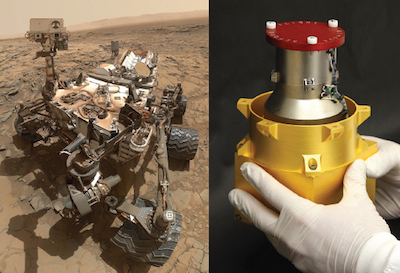Figure 8: The Curiosity rover (left) uses its radiation assessment detector (right) to record the surface radiation exposure on Mars. How can the data collected by Curiosity be used to make inferences about the typical levels of radiation a future Mars astronaut would be exposed to?image © NASA/JPL-Caltech/SwRI

Since it landed in August 2012, Curiosity has been using its radiation assessment detector to record the surface radiation exposure on Mars. A scientist in the future is analyzing this data and sees that there has been an average of 0.67 ± 0.29 millisieverts of radiation exposure per Martian day. (For comparison, sitting on the surface of Mars would be like taking approximately 35 chest X-rays every day.) This average is based on daily radiation exposure measurements recorded once every five Martian days over the past five Martian years for a total of 669 individual measurements.

Use this information to construct 50%, 80%, and 95% confidence intervals for the daily average radiation exposure on Mars. What are the subsample and population in this scenario? Can you identify any possible sources of sampling bias? (See our module Introduction to Inferential Statistics for a review of these terms.)

(Problem loosely based on Hassler et al., 2014)

### Solution

Because we are interested in knowing the daily average radiation on Mars, the population would be the total surface radiation measured every single day on Mars over the entire time that its current atmospheric conditions have existed and continue to exist. Observing this population is clearly impossible! Instead we must analyze a subsample to make inferences about the daily average radiation on Mars.

The subsample presented in this problem is the daily radiation exposure measured over five Martian years. This is a reasonably random subsample given that radiation exposure was recorded at equal intervals over several Martian years. Bias could have easily been introduced into the subsample if radiation exposure had only been recorded during certain seasons throughout the Martian year or if the instrument recording the radiation levels had not been properly calibrated. The fact that the subsample was collected over several years also helps account for solar fluctuations and other changes that might occur from one year to the next.

To construct our three confidence intervals, we can start by using the subsample mean (m = 0.67 mSV day-1) as a point estimation of the parameter mean. We can then calculate the margin of error in Excel using three values: the subsample size (N = 669), the subsample standard deviation (s = 0.29), and alpha. Because alpha is a function of the confidence level, we will need to compute a different value of alpha for each confidence interval:

• alpha = 1 – (50% ÷ 100%) = 0.5 at the 50% confidence level
• alpha = 1 – (80% ÷ 100%) = 0.2 at the 80% confidence level
• alpha = 1 – (95% ÷ 100%) = 0.05 at the 95% confidence level

In this problem we do not know any population parameters, so we will use the CONFIDENCE.T function in Excel. However, because the subsample size is so large (N = 669) both the CONFIDENCE.T and the CONFIDENCE.NORM functions will generate nearly the same confidence interval. Using the CONFIDENCE.T function in Excel calculates the margin of error:

• margin of error = 0.0076 mSV day-1 at the 50% confidence level
• margin of error = 0.014 mSV day-1 at the 80% confidence level
• margin of error = 0.022 mSV day-1 at the 95% confidence level

Taking these calculations together with our point estimation of the parameter mean gives us three confidence interval estimates for the daily average radiation exposure on Mars:

• 0.67 ± 0.0076 mSV day-1 at the 50% confidence level
• 0.67 ± 0.014 mSV day-1 at the 80% confidence level
• 0.67 ± 0.022 mSV day-1 at the 95% confidence level

We can show this graphically by plotting the confidence intervals as error bars on a bar graph (Figure 9). Notice how the size of the confidence interval changes for each confidence level. Out of the three confidence intervals we just constructed, the 50% confidence interval is the smallest but is also associated with the highest level of uncertainty. Conversely, the 95% confidence interval is the largest but is associated with the lowest level of uncertainty. In none of the cases can we know for certain where the true population mean lies – or whether the population mean falls within the confidence interval at all – but we can say that the interval estimate at the 95% confidence level are associated with a lower level of uncertainty than the interval estimates at lower confidence levels.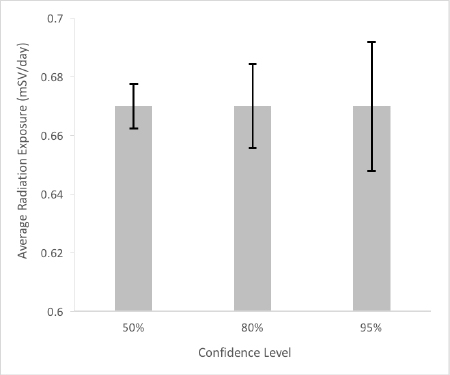Figure 9: Confidence intervals calculated at three different confidence levels for the mean radiation on Mars as measured by the Curiosity rover. Notice how the size of the confidence interval gets smaller as the level of uncertainty associated with the interval estimate becomes larger.

So what does this mean for our future Martian? Based on these calculations, the future scientists can move ahead with their spacesuit designs being fairly, though not absolutely, certain that the average daily radiation exposure on the surface of Mars – the true population mean in this scenario – falls somewhere within the interval of 0.67 ± 0.022 mSV day-1.

Like all of inferential statistics, confidence intervals are a useful tool that scientists can use to analyze and interpret their data. In particular, confidence intervals allow a researcher to make statements about how a single experiment relates to a larger underlying population or phenomenon. While Gosset’s t-distribution and Neyman’s confidence interval are common tools of statistical inference used in science, it is important to remember that there are numerous other methods for calculating interval estimates and drawing inferences from experimental subsamples. Just as the confidence interval can be a valuable technique in some situations, it can also be an unhelpful analytical tool in others. For example, a chemist trying to precisely measure the atomic mass of a new element would find no use for a 95% confidence interval that only provides a plausible range of values. In such a case, a much more stringent confidence level, or perhaps an entirely different statistical technique, would be more appropriate. Ultimately, as with all of statistical inference, it is up to the researcher to use techniques wisely and interpret data appropriately.

### Summary

Through history, important scientific advances have been made in connection with brewing beer. The module begins at the Guinness Brewery with the development of an important mathematical tool for inferential statistics. The focus of the module is confidence intervals, used when making statements about the relationship between a subsample and an entire population. Readers are shown how to construct and report a confidence interval. Topics include Student’s t-distribution, confidence level, critical value, and margin of error. Examples and a sample problem illustrate concepts introduced.

### Key Concepts

• Confidence intervals are a common type of inferential statistics estimate used in science. Starting with a subsample dataset, a scientist can construct a confidence interval that represents a plausible range for a population parameter while also indicating the level of error or uncertainty associated with the estimation.

• A confidence level represents the degree of uncertainty associated with a confidence interval. The higher the confidence level, the less uncertainty is associated with the confidence interval’s estimation of a population parameter. Although any value between 0% and 100% can theoretically be chosen, scientists typically calculate confidence intervals at the 90%, 95%, or 99% confidence level.

• Standard error is commonly encountered when using inferential statistics and is needed to calculate a confidence interval. It is important not to confuse standard error with standard deviation. Standard deviation is a descriptive statistic that represents the amount of variation in a sample, whereas standard error is an inferential statistic that represents a likely distance between a population parameter and a subsample statistic.

• ##### References
• Hassler, D.M., et al. (2013). Mars' surface radiation environment measured with the Mars Science Laboratory's Curiosity rover. Science, 343(6169): 1244797-1244797. Retrieved from: http://science.sciencemag.org/content/343/6169/1244797
• Neyman, J. (1937). Outline of a theory of statistical estimation based on the classical theory of probability. Philosophical Transactions of the Royal Society, 236: 333-380.
• Student. (1908). The probable error of a mean. Biometricka, 6(1): 1-25.

Liz Roth-Johnson, Ph.D. “Confidence Intervals” Visionlearning Vol. MAT-3 (6), 2016.

Top